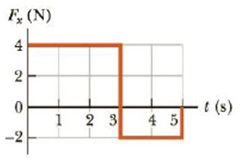Chapter 6, Problem 17P

Chapter
Section
Textbook Problem

The forces shown in the force vs. time diagram in Figure P6.17 act on a 1.5-kg particle. Find (a) the impulse for the interval from t = 0 to t = 3.0 s and (b) the impulse for the interval from t = 0 to t = 5.0 s. If the forces act on a 1.5-kg particle that is initially at rest, find the particle's speed (c) at t = 3.0 s and (d) at t = 5.0 s.Figure P6.17

(a)

To determine
Impulse of the force for t = 0 to t = 3.0s

Explanation

Given Info:

From the graph, width of the rectangle is 3.0s and height of the rectangle is 4.0N

The expression for impulse is

I=Arec

• I is the impulse,
• Arec is the area of rectangle,

From graph impulse is expressed as

I=wh

• w is the width of the rectangle,
• h is the height of the rectangle,

Substitute 3.0s for w and 4

(b)

To determine
The Impulse of the force for time period between 0 and 5.0 s

(c)

To determine
Final velocity of the particle at t=3.0s

(d)

To determine
Final velocity of the particle at t=5.0s

Still sussing out bartleby?

Check out a sample textbook solution.

See a sample solution

The Solution to Your Study Problems

Bartleby provides explanations to thousands of textbook problems written by our experts, many with advanced degrees!

Get Started

Find more solutions based on key concepts